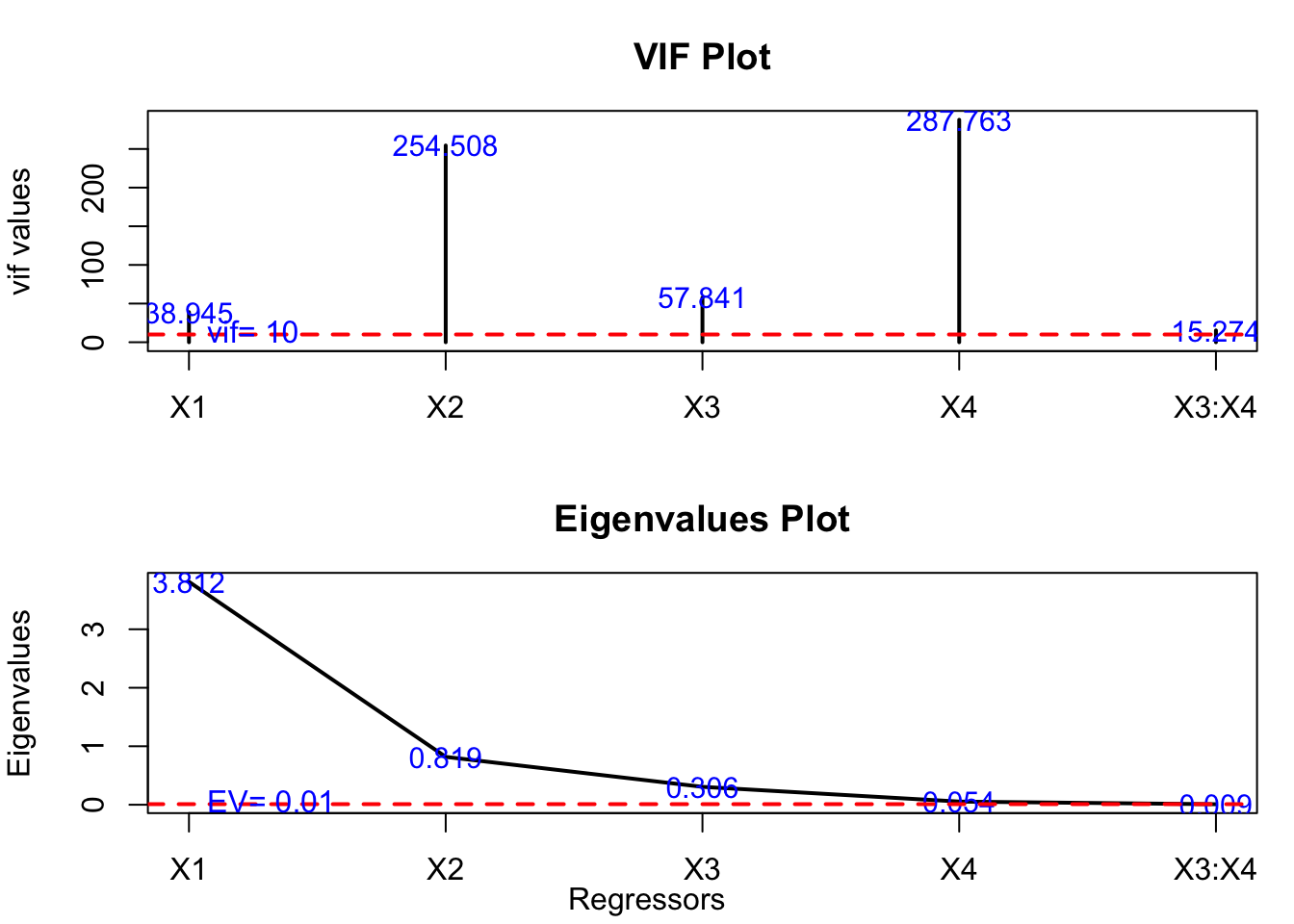There are various ways to get multicollinearity diagnostics in R. In the book I used the car package, but I occasionally run into difficulty when running different (e.g., older) R versions (which I occasionally need to do). I recently noticed the mctest package has VIF and a number of other diagnostics. However, it takes a y variable and a design matrix as input rather than a linear model object. For this reason I wrote a basic helper function that returns VIF from a linear model. It also has options for no intercept models (which can confuse vif functions) and to provide other statistics or a diagnostcs plot (all from mctest). It seems to work fine with lm() and glm() models and may well work with other model types.

 VIF <- function(linear.model, no.intercept=FALSE, all.diagnostics=FALSE, plot=FALSE) {
require(mctest)
if(no.intercept==FALSE) design.matrix <- model.matrix(linear.model)[,-1]
if(no.intercept==TRUE) design.matrix <- model.matrix(linear.model)
if(plot==TRUE) mc.plot(design.matrix,linear.model$model) if(all.diagnostics==FALSE) output <- imcdiag(design.matrix,linear.model$model, method='VIF')$idiags[,1] if(all.diagnostics==TRUE) output <- imcdiag(design.matrix,linear.model$model)
output
}

Using the default data from mctest here are some of the options. Note that 1/VIF is tolerance (though tolerance is also given by the all.diagnostics option).

library(mctest)
data(Hald)
mod.out <- lm(y~X1+X2+X3*X4, data=data.frame(Hald))

VIF(mod.out)
##        X1        X2        X3        X4     X3:X4
##  38.94527 254.50834  57.84057 287.76307  15.27360
1/VIF(mod.out)
##          X1          X2          X3          X4       X3:X4
## 0.025677062 0.003929144 0.017288903 0.003475081 0.065472434
VIF(mod.out, all.diagnostics=TRUE)
##
## Call:
## imcdiag(x = design.matrix, y = linear.model\$model)
##
##
## All Individual Multicollinearity Diagnostics Result
##
##            VIF    TOL       Wi       Fi Leamer    CVIF Klein
## X1     38.9453 0.0257  75.8905 113.8358 0.1602 -0.3558     0
## X2    254.5083 0.0039 507.0167 760.5250 0.0627 -2.3251     1
## X3     57.8406 0.0173 113.6811 170.5217 0.1315 -0.5284     1
## X4    287.7631 0.0035 573.5261 860.2892 0.0589 -2.6290     1
## X3:X4  15.2736 0.0655  28.5472  42.8208 0.2559 -0.1395     0
##
## 1 --> COLLINEARITY is detected
## 0 --> COLLINEARITY in not detected by the test
##
## X1 , X2 , X3 , X4 , X3:X4 , coefficient(s) are non-significant may be due to multicollinearity
##
## R-square of y on all x: 0.9824
##
## * use method argument to check which regressors may be the reason of collinearity
## ===================================
VIF(mod.out, plot=TRUE)##        X1        X2        X3        X4     X3:X4
##  38.94527 254.50834  57.84057 287.76307  15.27360
mod.out <- glm(y~0+X1+X2+X3*X4, data=data.frame(Hald))

VIF(mod.out, no.intercept=TRUE)
##        X1        X2        X3        X4     X3:X4
##  38.94527 254.50834  57.84057 287.76307  15.27360